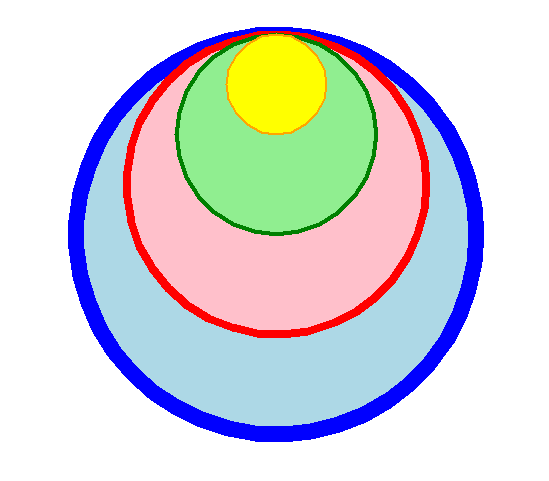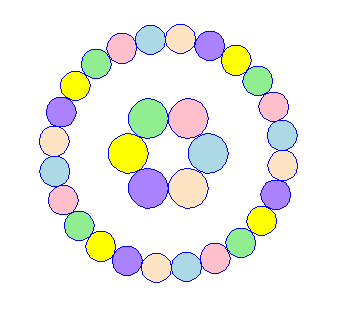# 10. Turtle circles

The code below draws circles.

## 10.1. Turtle Circles

The turtle syntax for drawing circles in below:
radius - radius; a positive number draws anticlockwise, a negative number draws clockwise.
extent - an angle; a number (or None for whole circle), which determines how many degrees of the circle is drawn; default None
steps - an integer (or None for a circle) which allows polygons to be drawn; default None
The center is radius units left of the turtle at right angles from its heading if the radius is positive.
The direction of the turtle is changed by the amount of extent.
The pensize increases the circle line inwards and outwards from the radius distance. So a pensize of 41 draws the circle 20 pixels inwards and 20 pixels outwards from the exact radius position. This is important for predicting circle edges with large pensizes.

## 10.2. Circles at a specified location

Adding a starting position, the centre of the circle, will provide some convenience.
The `draw_centered_circle` syntax is below:
draw_centered_circle(t, centre=(0, 0), radius=10, penw=1, penc='black', fillc=None)
t - the turtle object to draw the circle
centre - start position; default (0, 0)
penw - the pen width; default 1
penc - the pen color; a colorstring or a numeric color tuple (r, g, b,); default “black”
fillc - the fill color; a colorstring or a numeric color tuple (r, g, b,); default None
The `draw_centered_circle` definition code is below.
The code moves the turtle to the given centre, sets the angle to 0, moves forward the radius and sets the angle to 90, then draws the circle.
If there is a fill color given, then `begin_fill` and `end_fill` need to be used either side of the drawing.

Code Completion: draw_centered_circle definition

Complete the code for the `draw_centered_circle` definition by replacing the “XXX”s.

```def draw_centered_circle(t, centre=(0, 0), radius=10, penw=1, penc="black", fillc=None):
t.pu()
t.goto(XXX)
t.seth(XXX)
t.fd(XXX)
t.seth(XXX)
t.pensize(XXX)
t.pencolor(XXX)
t.pd()
if XXX is not None:
t.fillcolor(XXX)
t.begin_fill()
t.circle(XXX)
if XXX is not None:
t.end_fill()
```

Completed code for the `draw_centered_circle` definition.

```def draw_centered_circle(t, centre=(0, 0), radius=10, penw=1, penc="black", fillc=None):
t.pu()
t.goto(centre)
t.seth(0)
t.seth(90)
t.pensize(penw)
t.pencolor(penc)
t.pd()
if fillc is not None:
t.fillcolor(fillc)
t.begin_fill()
if fillc is not None:
t.end_fill()
```

## 10.3. Circles within circlesUse draw_centered_circle to draw circles within circles.
Use list indexing along with the lists given below.
centres = [(0, 0),(0, 50),(0, 100),(0, 150)]
radii = [200, 150, 100, 50]
pensizes = [16, 8, 4, 2]
pencolors = [“blue”, “red”, “green”, “orange”]
fillcolors = [“light blue”, “pink”, “light green”, “yellow”]

Code Completion: rectangle_steps_coloured definition

Complete the code to draw circles within circles, by replacing the XXXs.

```import turtle

def draw_centered_circle(t, centre=(0, 0), radius=10, penw=1, penc="black", fillc=None):
t.pu()
t.goto(centre)
t.seth(0)
t.seth(90)
t.pensize(penw)
t.pencolor(penc)
t.pd()
if fillc is not None:
t.fillcolor(fillc)
t.begin_fill()
if fillc is not None:
t.end_fill()

s = turtle.Screen()
s.bgcolor("white")
s.title("draw_centered_circle")
s.setup(width=800, height=600, startx=0, starty=0)

t = turtle.Turtle()
t.speed(0)
t.ht()

centres = [(0, 0),(0, 50),(0, 100),(0, 150)]
radii = [200, 150, 100, 50]
pensizes =  [16, 8, 4, 2]
pencolors = ["blue", "red", "green", "orange"]
fillcolors = ["light blue", "pink", "light green", "yellow"]

for i in range(XXX):
draw_centered_circle(t, centre=XXX, radius=XXX, penw=XXX, penc=XXX, fillc=XXX)

s.exitonclick()
```

Completed code to draw circles within circles.

```import turtle

def draw_centered_circle(t, centre=(0, 0), radius=10, penw=1, penc="black", fillc=None):
t.pu()
t.goto(centre)
t.seth(0)
t.seth(90)
t.pensize(penw)
t.pencolor(penc)
t.pd()
if fillc is not None:
t.fillcolor(fillc)
t.begin_fill()
if fillc is not None:
t.end_fill()

s = turtle.Screen()
s.bgcolor("white")
s.title("draw_centered_circle")
s.setup(width=800, height=600, startx=0, starty=0)

t = turtle.Turtle()
t.speed(0)
t.ht()

centres = [(0, 0),(0, 50),(0, 100),(0, 150)]
radii = [200, 150, 100, 50]
pensizes =  [16, 8, 4, 2]
pencolors = ["blue", "red", "green", "orange"]
fillcolors = ["light blue", "pink", "light green", "yellow"]

s.exitonclick()
```

Exercises

1. Modify the lists above to draw the concentric circles show:## 10.4. Rings of circles

The draw_ring_of_circles defintion draws a given number of circles in a circle shape.
In the code below, the draw_ring_of_circles definition requires the importing of 2 maths functions via: `from math import sin, radians`.
List multiplication is used in the line below in which the list is replicted to have 24 colors:
`colorlist=["light blue", "pink", "light green", "yellow", "MediumPurple1", "bisque"]*4`

Code Completion: rectangle_steps_coloured definition

Complete the code to draw a ring of circles as in the image by putitng in arguments to the draw_ring_of_circles calls, by replacing the XXXs.```import turtle

def draw_centered_circle(t, centre=(0, 0), radius=10, penw=1, penc="black", fillc=None):
t.pu()
t.goto(centre)
t.seth(0)
t.seth(90)
t.pensize(penw)
t.pencolor(penc)
t.pd()
if fillc is not None:
t.fillcolor(fillc)
t.begin_fill()
if fillc is not None:
t.end_fill()

def draw_ring_of_circles(t, centre, angle, size, sides, colors=None):
for i in range(sides):
t.pu()
t.goto(centre)
t.seth(angle + i*360//sides)
t.fd(size + circum_r)
dot_centre = t.pos()
if colors is None:
draw_centered_circle(t, centre=dot_centre, radius=circum_r, penw=1, penc="blue", fillc=None)
else:
dot_color = colors[i]
draw_centered_circle(t, centre=dot_centre, radius=circum_r, penw=1, penc="blue", fillc=dot_color)

s = turtle.Screen()
s.bgcolor("white")
s.title("draw_ring_of_circles")
s.setup(width=1000, height=1000, startx=0, starty=0)
s.tracer(0, 0)
s.colormode(255)

t = turtle.Turtle()
t.speed(0)
t.ht()

colorlist=["light blue", "pink", "light green", "yellow", "MediumPurple1", "bisque"]*4
draw_ring_of_circles(XXX)
draw_ring_of_circles(XXX)

s.update()
s.exitonclick()
```

Completed code to draw a ring of circles.

```import turtle

def draw_centered_circle(t, centre=(0, 0), radius=10, penw=1, penc="black", fillc=None):
t.pu()
t.goto(centre)
t.seth(0)
t.seth(90)
t.pensize(penw)
t.pencolor(penc)
t.pd()
if fillc is not None:
t.fillcolor(fillc)
t.begin_fill()
if fillc is not None:
t.end_fill()

def draw_ring_of_circles(t, centre, angle, size, sides, colors=None):
for i in range(sides):
t.pu()
t.goto(centre)
t.seth(angle + i*360//sides)
t.fd(size + circum_r)
dot_centre = t.pos()
if colors is None:
draw_centered_circle(t, centre=dot_centre, radius=circum_r, penw=1, penc="blue", fillc=None)
else:
dot_color = colors[i]
draw_centered_circle(t, centre=dot_centre, radius=circum_r, penw=1, penc="blue", fillc=dot_color)

s = turtle.Screen()
s.bgcolor("white")
s.title("draw_ring_of_circles")
s.setup(width=1000, height=1000, startx=0, starty=0)
s.tracer(0, 0)
s.colormode(255)

t = turtle.Turtle()
t.speed(0)
t.ht()

colorlist=["light blue", "pink", "light green", "yellow", "MediumPurple1", "bisque"]*4
draw_ring_of_circles(t, centre=(0, 0), angle=0, size=20, sides=6, colors=colorlist)
draw_ring_of_circles(t, centre=(0, 0), angle=9, size=100, sides=24, colors=colorlist)

s.update()
s.exitonclick()
```